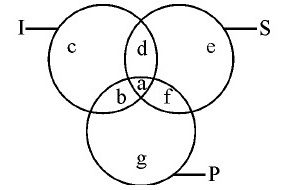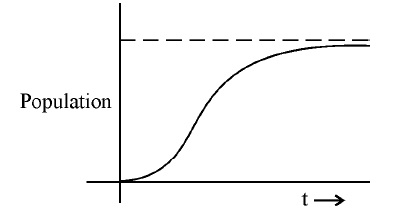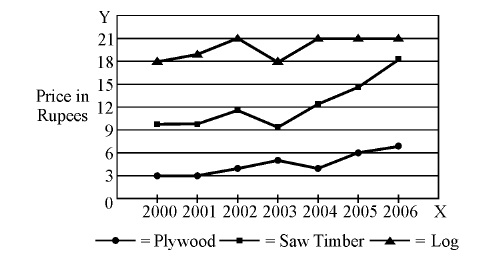UGC-NET/SET-PAPER-I(2010-JUNE) - MODEL PAPER-99
ONLINE TEST NET/SET PAPER-1
31. Which of the following is an example of circular argument ?
2 points
32. Lakshmana is a morally good person because
2 points
33. Two statements I and II given below are followed by two conclusions (a) and (b). Supposing the statements are true, which of the following conclusions can logically follow?
2 points
34. Certainty is
2 points
Questions from 35 to 36 are based on the following diagram in which there are three intersecting circles I, S and P where circle I stands for Indians, circle S stands for scientists and circle P for politicians. Different regions of the figure are lettered from a to g.
2 points36. The region which represents politicians who are Indians as well as scientists.
2 points
37. The population of a city is plotted as a function of time (years) in graphic form below:
2 pointsIn the following chart, the price of logs is shown in per cubic metre and that of Plywood and Saw Timber in per tonnes. Study the chart and answer the following questions 38, 39 and 40.
2 points39. What is the maximum percentage increase in price per cubic metre of log?
2 points
40. In which year the prices of two products increased and that of the third increased?
2 points